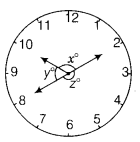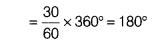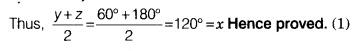# The picture given below shows the face of a clock. When the time is 10 : 10

The picture given below shows the face of a clock. When the time is 10 : 10, the hour hand and the minute hand form angle x and minute hand and second hand form angle z, whereas hour hand and second handForm angle y. Then, prove that x=y+z/2Angle formed by minute hand in 20 minAngle formed by second hand in 30 sec[angle formed by minute hand from 2 to 8] (1)
∴ z° = 180°
Now, y° = 360°-x°-z°
=> y°= 360°-120°-180° = 60°